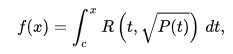# GlossariAquest glossari és una mostra dels camps més representatius en què Sofia Kovalevskaya va treballar. Les definicions han estat extretes dels recursos següents:

#### Abelian varieties

In mathematics, particularly in algebraic geometry, complex analysis and algebraic number theory, an abelian variety is a projective algebraic variety that is also an algebraic group, i.e., has a group law that can be defined by regular functions. Abelian varieties are at the same time among the most studied objects in algebraic geometry and indispensable tools for much research on other topics in algebraic geometry and number theory.

Citació: "Abelian variety" A: Wikipedia. Wikimedia Foundation, 2011. [en línia]. [Consulta: 18 setembre 2018]. Disponible a: <https://en.wikipedia.org/wiki/Abelian_variety>

Més informació:

#### Cauchy-Kovalevskaya theorem

In mathematics, the Cauchy–Kowalevski theorem (also written as the Cauchy–Kovalevskaya theorem) is the main local existence and uniqueness theorem for analytic partial differential equations associated with Cauchy initial value problems. A special case was proven by Augustin Cauchy (1842), and the full result by Sophie Kovalevskaya (1875).

Citació: "Cauchy–Kowalevski theorem" A: Wikipedia. Wikimedia Foundation, 2011. [en línia]. [Consulta: 18 setembre 2018]. Disponible a: <https://en.wikipedia.org/wiki/Cauchy%E2%80%93Kowalevski_theorem>

Més informació:

#### Elliptic integrals

In integral calculus, elliptic integrals originally arose in connection with the problem of giving the arc length of an ellipse. They were first studied by Giulio Fagnano and Leonhard Euler (c. 1750). Modern mathematics defines an "elliptic integral" as any function f which can be expressed in the formwhere R is a rational function of its two arguments, P is a polynomial of degree 3 or 4 with no repeated roots, and c is a constant.

In general, integrals in this form cannot be expressed in terms of elementary functions. Exceptions to this general rule are when P has repeated roots, or when R(x,y) contains no odd powers of y. However, with the appropriate reduction formula, every elliptic integral can be brought into a form that involves integrals over rational functions and the three Legendre canonical forms (i.e. the elliptic integrals of the first, second and third kind).

Besides the Legendre form given below, the elliptic integrals may also be expressed in Carlson symmetric form. Additional insight into the theory of the elliptic integral may be gained through the study of the Schwarz–Christoffel mapping. Historically, elliptic functions were discovered as inverse functions of elliptic integrals.

Citació: "Elliptic integrals" A: Wikipedia. Wikimedia Foundation, 2011. [en línia]. [Consulta: 18 setembre 2018]. Disponible a: <https://en.wikipedia.org/wiki/Mathematical_analysis>

Més informació:

#### Mathematical Analysis

Mathematical analysis is the branch of mathematics dealing with limits and related theories, such as differentiation, integration, measure, infinite series, and analytic functions.

These theories are usually studied in the context of real and complex numbers and functions. Analysis evolved from calculus, which involves the elementary concepts and techniques of analysis. Analysis may be distinguished from geometry; however, it can be applied to any space of mathematical objects that has a definition of nearness (a topological space) or specific distances between objects (a metric space).

Citació: "Mathematical analysis" A: Wikipedia. Wikimedia Foundation, 2011. [en línia]. [Consulta: 18 setembre 2018]. Disponible a: <https://en.wikipedia.org/wiki/Mathematical_analysis>

Més informació:

#### Partial differential equation

In mathematics, a partial differential equation (PDE) is a differential equation that contains unknown multivariable functions and their partial derivatives. PDEs are used to formulate problems involving functions of several variables, and are either solved by hand, or used to create a computer model. A special case is ordinary differential equations (ODEs), which deal with functions of a single variable and their derivatives.

PDEs can be used to describe a wide variety of phenomena such as sound, heat, electrostatics, electrodynamics, fluid dynamics, elasticity, or quantum mechanics. These seemingly distinct physical phenomena can be formalised similarly in terms of PDEs. Just as ordinary differential equations often model one-dimensional dynamical systems, partial differential equations often model multidimensional systems. PDEs find their generalisation in stochastic partial differential equations.

Citació: "Partial differential equation" A: Wikipedia. Wikimedia Foundation, 2011. [en línia]. [Consulta: 18 setembre 2018]. Disponible a: <https://en.wikipedia.org/wiki/Partial_differential_equation>

Més informació:

#### Rigid body dynamics

Rigid-body dynamics studies the movement of systems of interconnected bodies under the action of external forces. The assumption that the bodies are rigid, which means that they do not deform under the action of applied forces, simplifies the analysis by reducing the parameters that describe the configuration of the system to the translation and rotation of reference frames attached to each body. This excludes bodies that display fluid, highly elastic, and plastic behavior.

The dynamics of a rigid body system is described by the laws of kinematics and by the application of Newton's second law (kinetics) or their derivative form Lagrangian mechanics. The solution of these equations of motion provides a description of the position, the motion and the acceleration of the individual components of the system and overall the system itself, as a function of time. The formulation and solution of rigid body dynamics is an important tool in the computer simulation of mechanical systems.

Citació: "Rigid-body dynamics" A: Wikipedia. Wikimedia Foundation, 2011. [en línia]. [Consulta: 18 setembre 2018]. Disponible a: <https://en.wikipedia.org/wiki/Rigid_body_dynamics>

Més informació:

#### Theta function

In mathematics, theta functions are special functions of several complex variables. They are important in many areas, including the theories of Abelian varieties and moduli spaces, and of quadratic forms. They have also been applied to soliton theory. When generalized to a Grassmann algebra, they also appear in quantum field theory.

The most common form of theta function is that occurring in the theory of elliptic functions. With respect to one of the complex variables (conventionally called z), a theta function has a property expressing its behavior with respect to the addition of a period of the associated elliptic functions, making it a quasiperiodic function. In the abstract theory this comes from a line bundle condition of descent.

Citació: "Theta function" A: Wikipedia. Wikimedia Foundation, 2011. [en línia]. [Consulta: 18 setembre 2018]. Disponible a: <https://en.wikipedia.org/wiki/Theta_function>

Més informació: